International
Tables for
Crystallography
Volume D
Physical properties of crystals
Edited by A. Authier

International Tables for Crystallography (2006). Vol. D, ch. 1.1, p. 14

## Section 1.1.4.5.3.2. Tensors of higher rank

A. Authiera*

aInstitut de Minéralogie et de la Physique des Milieux Condensés, Bâtiment 7, 140 rue de Lourmel, 75015 Paris, France
Correspondence e-mail: aauthier@wanadoo.fr

#### 1.1.4.5.3.2. Tensors of higher rank

| top | pdf |

If the rank of the tensor is higher than 2, the tensor may be antisymmetric with respect to the indices of one or several couples of indices.

 (i) Tensors of rank 3 antisymmetric with respect to every couple of indices. A trilinear form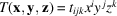is said to be antisymmetric if it satifies the relations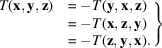Tensor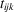has 27 components. It is found that all of them are equal to zero, except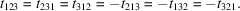The three-times contracted product with the permutations tensor (Section 1.1.3.7.2),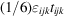, is a pseudoscalar or axial scalar. It is not a usual scalar: the sign of this product changes when one changes the hand of the reference axes, change of basis represented by the matrix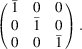Form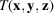can also be written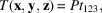where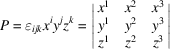is the triple scalar product of the three vectors x, y, z: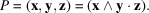It is also a pseudoscalar. The permutation tensor is not a real tensor of rank 3: if the hand of the axes is changed, the sign of P also changes; P is therefore not a trilinear form. Another example of a pseudoscalar is given by the rotatory power of an optically active medium, which is expressed through the relation (see Section 1.6.5.4)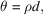where θ is the rotation angle of the light wave, d the distance traversed in the material and ρ is a pseudoscalar: if one takes the mirror image of this medium, the sign of the rotation of the light wave also changes. (ii) Tensor of rank 3 antisymmetric with respect to one couple of indices. Let us consider a trilinear form such that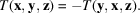Its components satisfy the relation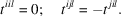The twice contracted product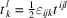is an axial tensor of rank 2 whose components are the independent components of the antisymmetric tensor of rank 3,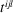.

#### Examples

 (1) Hall constant. The Hall effect is observed in semiconductors. If one takes a semiconductor crystal and applies a magnetic induction B and at the same time imposes a current density j at right angles to it, one observes an electric field E at right angles to the other two fields (see Section 1.8.3.4). The expression for the field can be written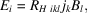where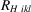is the Hall constant, which is a tensor of rank 3. However, because the direction of the current density is imposed by the physical law (the set of vectors B, j, E constitutes a right-handed frame), one has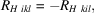which shows thatis an antisymmetric (axial) tensor of rank 3. As can be seen from its physical properties, only the components such that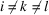are different from zero. These are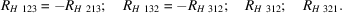(2) Optical rotation. The gyration tensor used to describe the property of optical rotation presented by gyrotropic materials (see Section 1.6.5.4) is an axial tensor of rank 2, which is actually an antisymmetric tensor of rank 3. (3) Acoustic activity. The acoustic gyrotropic tensor describes the rotation of the polarization plane of a transverse acoustic wave propagating along the acoustic axis (see for instance Kumaraswamy & Krishnamurthy, 1980). The elastic constants may be expanded as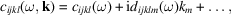where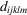is a fifth-rank tensor. Time-reversal invariance requires that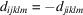, which shows that it is an antisymmetric (axial) tensor.

### References

Kumaraswamy, K. & Krishnamurthy, N. (1980). The acoustic gyrotropic tensor in crystals. Acta Cryst. A36, 760–762.